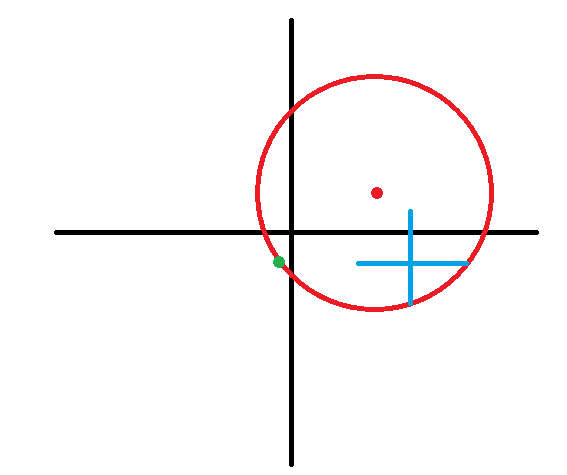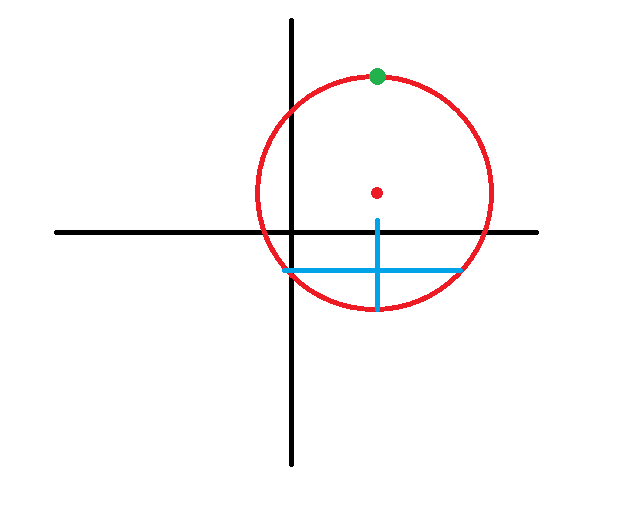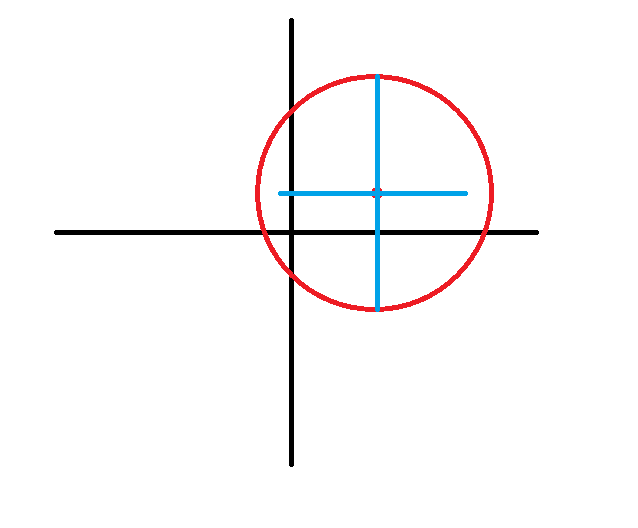Background calibration for magnetometers, both gain and offset errors are removed (hard/soft iron).

Magnetometers are notoriously bad when uncalibrated. There are both gain and offset errors where the offset errors. Gain errors can often be removed by the magnetometer itself, many got options to apply a bias magnetic field of known strength, which can be measured and used to calculate gain errors. However the most significant errors are the offset errors, they can be huge. The offset on an axis can be so large that it will always measure the Earth magnetic field in one direction, which pretty much ruins your day if you want to calculate where the north is.

Just measuring this offset (output its measurement data to your PC, rotate it a bit, see what the offset is) already helps alot. However I consider automatic calibration algorithms nice, how practical they are is a whole different story.

# Algorithms

Before I made this I looked a bit around online regarding automatic calibration algorithms for magnetometers. The vast majority are besides very computational intensive also really confusing to me, way too much vector algebra. Then we got those who get the offset simply by averaging their measurements, where each measurement gets a weight factor depending on the amount of rotation measured by the gyroscope. The idea is that if you have for example a quadrocopter it will generally point same amount of time in each direction, and if you use the gyroscope data it will prevent the offset calculations from going horribly wrong when it is pointed one direction for a long time. I am sure it works, but I do not consider it a really neat solution.

Another option is measuring the 'extremes' reported by each axis. If the magnetometer has been in use for a while, and on the x-axis one extreme is -80, and the other one is 120, the offset is obviously 20. And this way you can also immediatly compensate for gain errors. However there is one serious shortcome of this method: if due to whatever reason you make an incorrect measurement of for example 200, your offset and gain estimate is wrong. You can of course lower the impact of this by taking the average of the old value and new value, so one incorrect measurement can only have a limitted impact. However the issue remains that your extremes can only become more 'extreme'. There is no way to use new measurements to lower the value of your calculated extreme value.

## Used algorithm

And that is where this algorithm comes into play. In principle it is based on calculating the extreme values of the magnetometer. If a new extreme is found, it will change the old one with a certain gain. However it adds one extra step: it takes into account that when two axes are close to their zero value (compensated for offset), the third one must be at an extreme value. It will then use this data to update the value of its stored extremes: if the measured value is larger than its current offset it will update its positive extreme value, if it is smaller it will update its negative extreme value. That immediatly brings us to the limitation of the algorithm, when the current estimate of the offset is so bad it is outside the actual values the magnetometer can reach, it may not be able to find the actual extremes.

## 2D example

I hope that was a little bit clear, but it is probably easier to visualize with a 2D example. In the following picture the red circle shows the values our magnetometric sensor can achieve if the world was 2D, with in the center the dot representing its offset. The blue lines connect its current values for its extremes (which are obviously wrong), and the green dot is a new measurement.Since the y-axis is zero for this measurement, it will know this is a new extreme value. The measured value is smaller than its offset estimate (the point where the lines of the extremes cross), so it is a new negative extreme for the x-axis. So it will moves its negative extreme value a bit to this new measurement (how fast depends on the gain settings), but lets assume it got a whole bunch of measurements on this spot, so its negative extreme is now at this measurement location.

The next figure gives the resulting situation, and after a while the magnetometer gets to a spot where its x-axis value is zero, and it gets a new positive extreme measurement for the y-axis.The same thing happens again, and it adjusts its y-axis extremes, and also gets a new offset value for this axis. Making the new result:Now it only needs some measurements to get the gain on its x-axis correct, and it is fully calibrated. When the offset changes for whatever reason, it will automatically use its new measurements to get new estimates of its extremes, and use that to calculate its gain and offset. Besides this algorithm it also directly updates the extreme when it literally finds a new extreme value (still with small steps). So if in the previous example it immediatly gets to the point completely at the leftmost point of the circle it will use this to update the minimum extreme of the x-axis, despite that the y-axis data is not on its offset estimate.

In this example the initial extremes were too small, and an algorithm that only checks if the current measurement value is larger than its stored extreme value would also work fine. However the difference is that this also works if the initial extremes were too large.

# Usage of the library

The library does not use any knowledge about how large the signal is supposed to be, it does not matter if they got a size of +/- 0.001, or +/- 10,000. This results in a problem since it really has no clue about the initial estimates it should start with, and for this reason the first 100 measurement points it only stores the extreme values found, and its output is identical to its input. For good results you want to rotate the sensor alot during these initial points, but really it is only useful when running it the first time to get a global estimate of the extremes.

For normal usage it is best to use the setExtremes function in the beginning to supply the filter with its starting points. When setExtremes is called it will disable those first 100 measurement points, and will function normally from the start. It is probably ideal to at the end of your program let the mbed write the latest extreme values of the filter (getExtremes) to a non-volatile memory, such as the localFileSystem. Then at the start of your program you get these values again, so it will always use the most accurate initial conditions.

The output of the filter is the magnetic vector, compensated for gain and offset, normalized to a length of one. Generally the length of the magnetic vector is not used for anything, so this should not be a problem. Do not this normalization is not absolute: it just uses its gain and offset estimates to normalize to a length of roughly one (in practise a bit more than one), but it does not actually normalize the vector to have exactly a length of one.

Because the filter only uses fairly simple calculations: multiplications, additions and comparisons mostly, and not computational intensive stuff such a sine and square root calculations, it is pretty lightweight. I think execution takes around 10us, but I will update that value when I check that again.